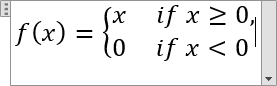# How to insert an equation with a system of linear equations or linear system

Word
This tip shows how to add an equation with a system of linear equations (or linear system) – a collection of two or more linear equations involving the same set of variables.

How to add an equation in your document, see Working with Microsoft Equation.

To insert a system of linear equations, do the following:

## In the Professional format:

1.   In your own equation, enter f (x)=.

2.   Under Equation Tools, on the Design tab, in the Structures group, click the Bracket button: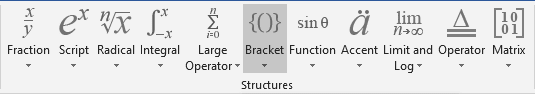In the Bracket list, under Cases and Stacks, choose Cases (Two Conditions):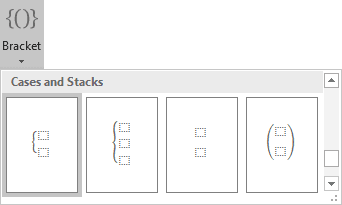3.   In every box, enter the appropriate formula, for example: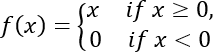To insert a new line for this system of linear equations, just click Enter: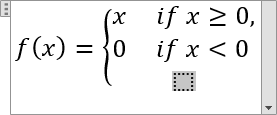## In the Linear format:

1.   In your own equation, enter f (x) = {.

2.   Enter \eqarray to start a system of linear equations. Then you enter a space key, this linear formula transformed to the professional format: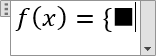3.   In the brackets, enter formulas with symbols @ that divide rows in the linear system: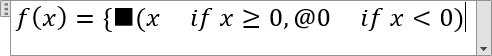4.   Enter \close to end the system of linear equations: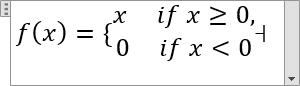Then you enter a space key, the formula transformed to the professional format: